###### 1. Differentiate between asset allocation and diversification?

Asset allocation emphasizes on investing into various assets classes while diversification emphasizes on selecting the securities to be included into a given asset class. Hence, investors can use asset allocation as a way to diversify their investments among asset classes.

###### 2. What is the difference between realized capital gains and unrealized capital gain?

The realized capital gains are those which results from the sale of any securities in the portfolio. The unrealized capital gains are the paper profits resulted from appreciation in market value of the portfolio over two periods of time.

NUMERICAL PROBLEMS

###### 3. Assume that Portfolio A has Treynor’s measure of 2.36 and that of the market is 3.10. Do you think that Portfolio A has outperformed the market? Why or why not?

SOLUTION

The Portfolio A has not outperformed the market on risk-adjusted basis, because its Treynor measure (2.36) is less than that of the market (3.10).

###### 4. A portfolio M has average return of 20 percent, beta of the portfolio is 1.5, and standard deviation 8 percent. If risk-free rate is 6 percent, calculate Sharpe’s measure of portfolio performance.

SOLUTION

Given:

Portfolio return, E(Rp) = 20%

Portfolio beta (p) = 1.5

Portfolio standard deviation (P) = 8%

Risk-free rate (Rf) = 6%

Sharpe’s measure for the portfolio is given by:

Sp  = E(Rp) – Rf / (P) =  20% -6% /8% = = 1.75

###### 5. A portfolio has average return of 12 percent with a standard deviation of 6 percent. If the risk-free rate is 5 percent, what is the Sharpe’s measure of the portfolio?

SOLUTION

Given:

Portfolio return (Rp) = 12%

Portfolio standard deviation (P) = 6%

Risk-free rate (RF) = 5%

Sharpe’s measure for the portfolio is given by:

Sp  =      Rp – R/  (P)

=  12% – 5% / 6%

= 1.17

###### 6. A portfolio has Sharpe’s index of 1.2 while that of the market portfolio is 1.3. Do you think that the portfolio has outperformed the market? Why?

SOLUTION

No, the portfolio has not outperformed the market because Sharpe’s measure of portfolio (1.2) is lower than that of market portfolio (1.3)

###### 1. Differentiate Sharpe’s measure of portfolio performance from Treynor’s measure.

Sharpe’s measure of portfolio performance, developed by William F. Sharpe, provides a portfolio performance index that compares a portfolio’s risk premium to the total risk associated with the portfolio A portfolio’s risk premium is defined as the excess of total rate of return on portfolio above risk free rate of return. The portfolio’s total risk is measured by the standard deviation of portfolio’s return. However, Treynor’s measure of portfolio performance, developed by Jack L. Treynor, is different from the Sharpe’s measure in consideration of portfolio risk. This measure provides a portfolio performance index that compares a portfolio’s risk premium to the systematic or non-diversifiable risk associated with the portfolio. The basic assumption underlying the consideration of systematic risk, as in the case of CAPM, is that unsystematic risk is not relevant to a well diversified portfolio and hence they are diversified away. The systematic risk is measured by an index of systematic risk called beta.

###### 2. What do you mean by asset allocation? What role does it play in constructing an investment portfolio? Explain.

Before selecting any category of securities to be included into the portfolio, the investor must develop a sound asset allocation scheme. An asset allocation is an investment plan that attempts to balance risk versus reward by allocating an investment portfolio across different assets categories that are consistent to risk tolerance, goals and investment time frame of investors. In other words, it involves dividing an investment portfolio among different asset categories such as stocks, bonds, short-term money market securities, foreign securities and so on. An efficient asset allocation plays important role in constructing an investment portfolio. They are as follows:

Capital preservation: An efficient asset allocation plan focuses on capital preservation. It is always based on the analysis of external economic forces and investor’s own personal situations such as size of income, savings and spending pattern, investor’s tax situation, return expectations, and risk tolerance. These analyses help in preserving capital.

Risk reduction: Market conditions that cause one asset category to do well often cause another asset category to have average or poor performance. By investing in more than one asset category, the investor will be able to reduce the risk, and improve portfolio’s overall investment returns.

###### 3. What is active portfolio management? Will it result in superior returns? Explain.

An investor can either adopt the passive or active portfolio management strategy. Active portfolio management is based on the belief that a specific style of management can produce returns that beat the market. Market timing is an extreme example of active portfolio management. This approach assumes that it is possible to anticipate the market movements based on factors such as economic conditions, interest rate trends and other technical indicators. The passive and active portfolio management differs in how the portfolio manager utilizes investments held in the portfolio over time. Active portfolio management focuses on outperforming the market compared to a specific benchmark, while passive portfolio management aims to copy the investment holdings of a particular market index.

Studies have shown that active management boost the potential for greater returns than those achieved simply copying the stocks or other securities listed on a particular index. Thus, we follow the ideas that active portfolio management has greater scope to improve the chance of earning super normal returns because portfolio analysis is at the heart of active portfolio management. The active portfolio management calls for assessing the portfolio performance regularly and using the information from the assessment to revise the portfolio as per the necessity. Thus, the active portfolio management usually results in the superior returns

###### 4. Why is Jensen’s measure generally preferred over the Sharpe’s and Treynor’s measures? Explain.

Jensen’s measure of portfolio performance is more preferable to Sharpe’s and. Treynor’s measure. Jensen’s measure does not require a separate comparison to the market. It automatically takes into account the market performance using the market adjusted CAPM return. Positive value of Jensen´s alpha simply indicates that the portfolio outperforms the market on a risk-adjusted basis. Its reliance only on non-diversifiable risk and its inclusion of both risk adjusted and market-adjusted return make it more preferable to other measures of portfolio performance.

###### 5. Assume that the risk free rate is currently 7 percent. The accompanying table provides you the risk and return data for the ABC fund and the market portfolio during the year just ended.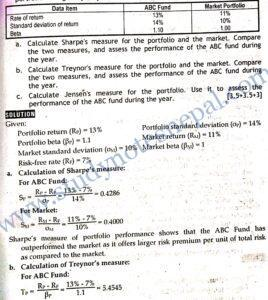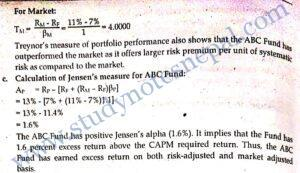1. Suppose your portfolio has 13 percent return, while the market return is 10 percent, and the risk-free rate is 6 percent. Your portfolio beta equals to 1.5. What is the Jensen’s alpha of your portfolio? Does your portfolio outperform the market portfolio on risk-adjusted basis? Explain.

SOLUTION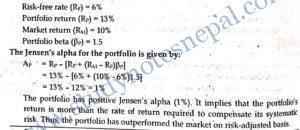2. Based on current dividend yields and capital gains, the rates of return on portfolios A and B are 15 percent and 20 percent, respectively. The beta of A is 0.8, while that of B is 1.2. The T-bill rate is currently yielding 5 percent, whereas the expected rate of return of the NEPSE index is 18 percent. The standard deviation of portfolio A is 10 percent, portfolio B is 19 percent, and that of the NEPSE index is 17 percent.

1. Evaluate the Portfolio A and Portfolio B based on Sharpe’s measure.
2. Evaluate the Portfolio A and Portfolio B based on Treynor’s measure.
3. Evaluate the Portfolio A and Portfolio B based on Jensen’s measure.

SOLUTION

Given:

Return on portfolio A (RA) = 15%

Return on portfolio B (RB) = 20%

Beta of portfolio A (A) = 0.8

Beta of portfolio B (B) = 1.2

Standard deviation of portfolio A (A) = 10%

Standard deviation of portfolio B (B) = 19%

Market return (RM) = 18%

Market standard deviation (M) = 17%

Risk-free rate (RF) = 5%3. You want to evaluate portfolio A, consisting entirely of common stocks using both the Treynor’s and Sharpe’s measures of portfolio performance. The following table provides the average annual-rate of return from portfolio A, the market portfolio, and Treasury bills during the past 8 years.

 Average annual rate of return Standard deviation of return Beta Portfolio A 12% 20% 0.60 Market portfolio 14 15 1.00 T-bills 8
1. Calculate the Sharpe’s, Treynor’s, and Jensen’s measures for both portfolios A and the market. Briefly explain whether portfolio A underperformed, equaled, or outperformed the market on a risk adjusted basis using all three measures.
2. Based on the performance of portfolio A relative to the market calculated in part (a), briefly explain the reason for the conflicting results when using the three methods.

SOLUTION

Given: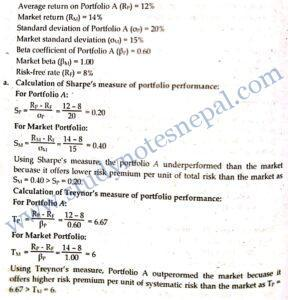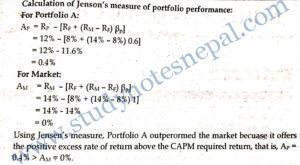These measures of portfolio performance differ with each other in terms of methodology and assumption. Sharpe’s measure takes into account total risk measured by standard deviation of portfolio while Treynor’s and Jensen’s measure considers systematic risk measured by beta. If a portfolio is perfectly diversified and its only component of risk is systematic risk all these methods give consistent ranking. The difference in ranking observed according to these methods is attributed to the level of diversification of the portfolio. Though a portfolio has higher total risk, its level of systematic risk may be lower due to the diversification effect. Thus, sometimes Treynor’s and Jensen’s measures give different raking of the portfolio than Sharpe’s measure. If a portfolio is poorly diversified, it would show better performance than the market according to Treynor’s and Jensen’s measure while it would show poor performance according to Sharpe’s measure. Thus, we can conclude that the Portfolio A is poorly diversified.